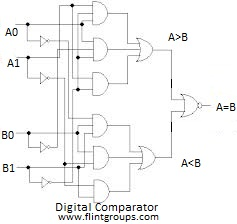# What is Digital Comparator?

What is Digital Comparator?
Digital Comparator (or) Binary Comparator is a Combinational Circuit made up from AND, NOR and NOT gates Which is used to compare Digital Signals.Suppose if we have two numbers A and B.The output of the Comparators are A greater B (A>B),A is equal to B (A=B) and A less than B (A<B).Digital Comparator is used in digital systems such as execution of conditional statements in microprocessors and microcontrollers.Consider A and B are two digit numbers.it canbe specified as

A=A1A0
B=B1B0
Equality
If the two Numbers are equal then  A1= B1 and A0= B0 and it is logically expressed as
Xi=AiBi+(AiBi)'.

Here Xi=1 only if the pair of bits in position i are equal that means  Ai and Bi are equal Where (i=0,1,2,3).

The output Variable
(A=B)=x1x0 will be 1.when all digits of A and B are Same.

Inequality

In order to manually determine  A is Greater Than or Less than B,the Relative magnitudes of pairs digits from most significant bit (MSB) is examined.until a pir of unequal digits is reached.if the corresponding bit of A is 1 and that of B is 0 then we conclude that A>B.

This sequential comparison can be expressed logically as:
(A>B) = A1B'1 + XiA0B'0
(A<B) = A'1B1 + XiA'0B0
The Truth Table  is Shown below.The Circuit Diagram is